#Pre-Algebra Worksheets

## Monomials and Polynomials Worksheets

Here is a graphic preview for all of the Monomials and Polynomials Worksheets. You can select different variables to customize these Monomials and Polynomials Worksheets for your needs. The Monomials and Polynomials Worksheets are randomly created and will never repeat so you have an endless supply of quality Monomials and Polynomials Worksheets to use in the classroom or at home. Our Monomials and Polynomials Worksheets are free to download, easy to use, and very flexible.

Click here for a Detailed Description of all the Monomials and Polynomials Worksheets.

## Quick Link for All Monomials and Polynomials Worksheets

Click the image to be taken to that Monomials and Polynomials Worksheets.

##### Identifying the Type ofMonomials and PolynomialsWorksheets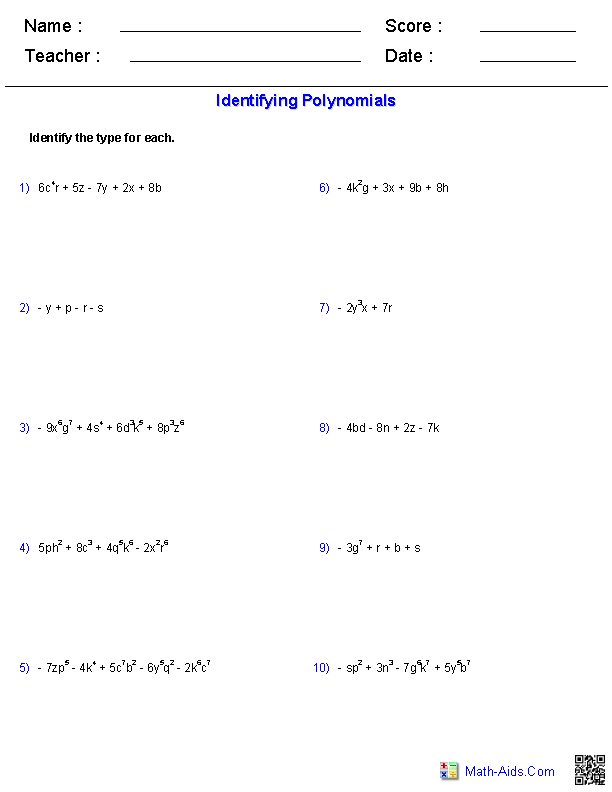##### Identifying the Degree ofMonomials and PolynomialsWorksheets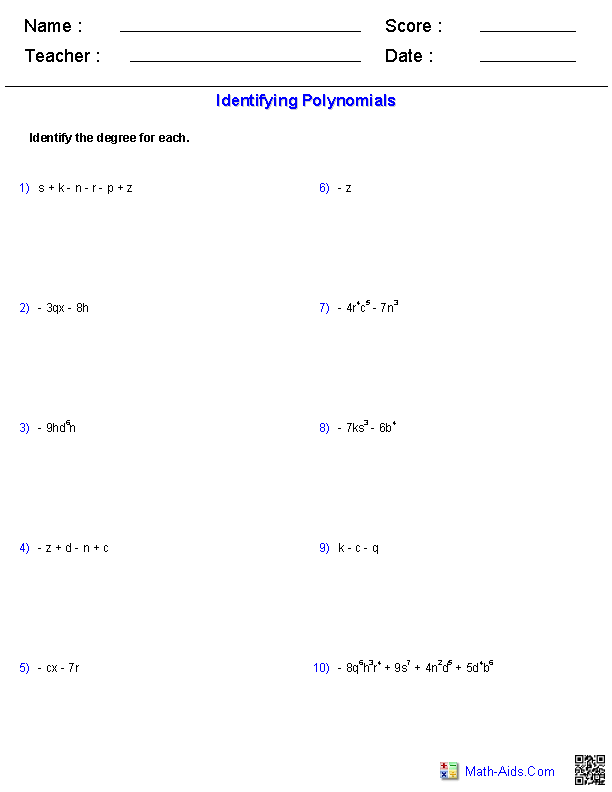##### FactoringMonomials and PolynomialsWorksheets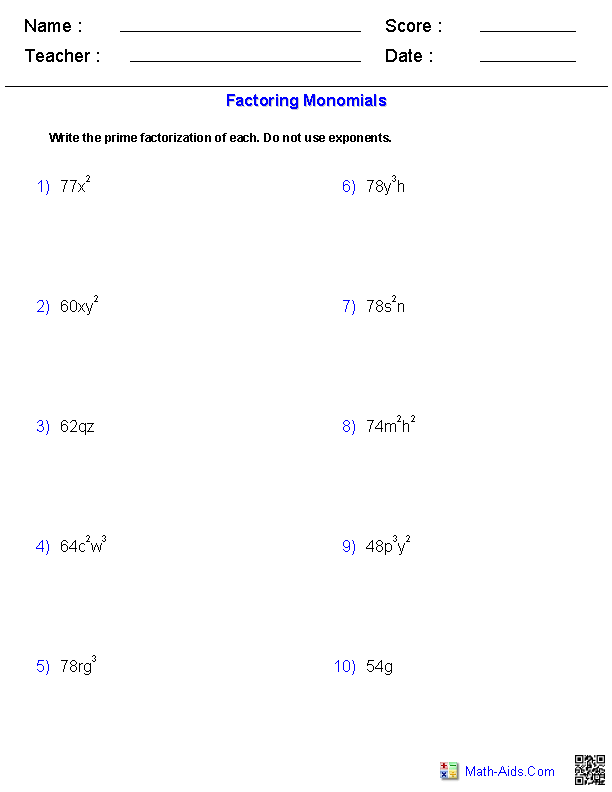##### Adding and SubtractingMonomials and PolynomialsWorksheets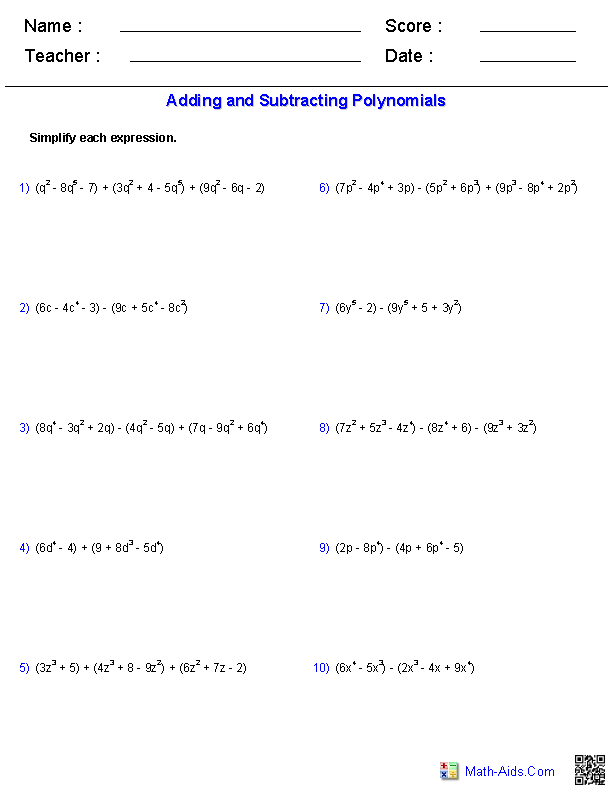##### Multiplying a Monomialand a PolynomialWorksheets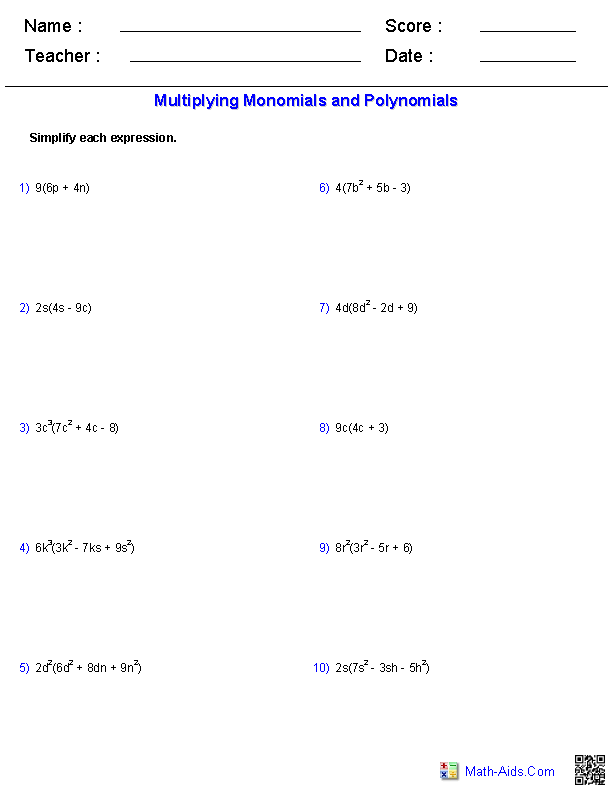##### Multiplying BinomialsMonomials and Polynomials Worksheets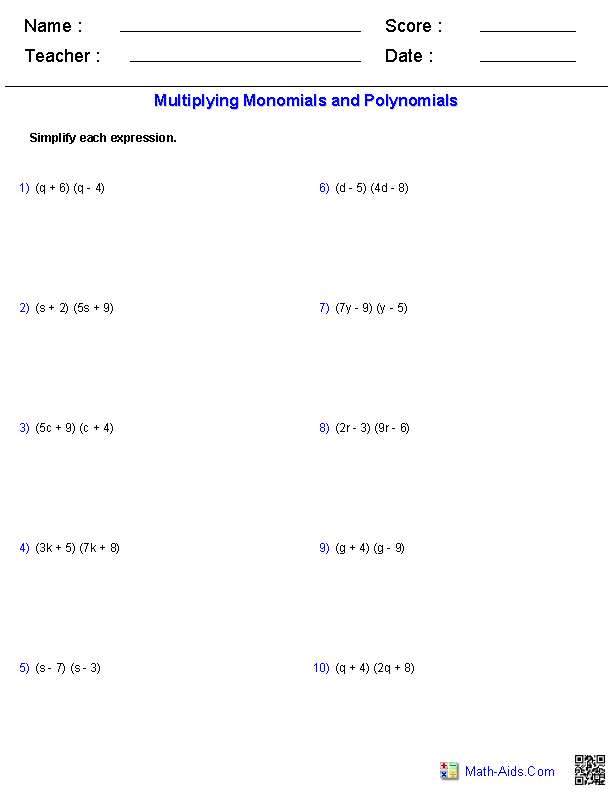##### Multiplying Monomials Monomials and Polynomials Worksheets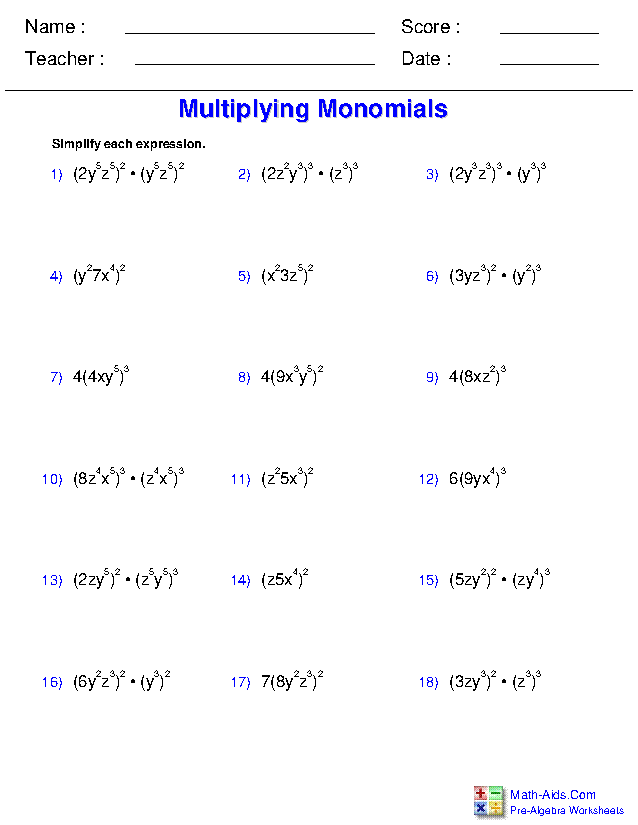Recommended Videos

## Description For All Monomials and Polynomials Worksheets

Identifying the Type of Monomials and Polynomials Worksheets
This monomial and polynomial worksheet will produce problems for identifying the type of monomials and polynomials equations. You may select which type of monomials and polynomials equations to use. This monomial and polynomial worksheet will produce ten problems per page. This monomials worksheet is a good resource for students in the 5th Grade, 6th Grade, 7th Grade, and 8th Grade.

Identifying the Degree of Monomials and Polynomials Worksheets
This monomial and polynomial worksheet will produce problems for identifying the degree of monomials and polynomials equations. You may select which type of monomials and polynomials problem to use. This monomial and polynomial worksheet will produce ten problems per page. This monomials worksheet is a good resource for students in the 5th Grade, 6th Grade, 7th Grade, and 8th Grade.

Factoring Monomials Worksheets
This monomials worksheet will produce problems for factoring monomials. You may select which type of monomial problems to use, the type of factorization to perform, and the range of numbers to use in the constants. This monomial worksheet will produce ten problems per page. This monomials worksheet is a good resource for students in the 5th Grade, 6th Grade, 7th Grade, and 8th Grade.

This polynomial worksheet will produce problems for adding and subtracting polynomials. You may select which type of polynomials problem to use and the range of numbers to use as the constants. This polynomial worksheet will produce ten problems per page. This monomial worksheet will produce ten problems per page. This monomials worksheet is a good resource for students in the 5th Grade, 6th Grade, 7th Grade, and 8th Grade.

Multiplying a Monomial and a Polynomial Worksheets
This monomial and polynomial worksheet will produce problems for multiplying monomials and polynomials. You may select which type of monomials and polynomials problem to use. This monomial and polynomial worksheet will produce ten problems per page. This monomial and polynomial worksheet will produce ten problems per page. This monomials worksheet is a good resource for students in the 5th Grade, 6th Grade, 7th Grade, and 8th Grade.

Multiplying Binomials Worksheets
This monomial and polynomial worksheet will produce problems for multiplying binomials. You may select which type of binomials problem to use. This monomial and polynomial worksheet will produce ten problems per page. This monomials worksheet is a good resource for students in the 5th Grade, 6th Grade, 7th Grade, and 8th Grade.

Multiplying Monomials Worksheets
This monomial and polynomial worksheet will produce problems for multiplying monomials. You may select the number of problems, the types of expressions, as well as the parities of both the powers and constants. This monomials worksheet is a good resource for students in the 5th Grade, 6th Grade, 7th Grade, and 8th Grade.